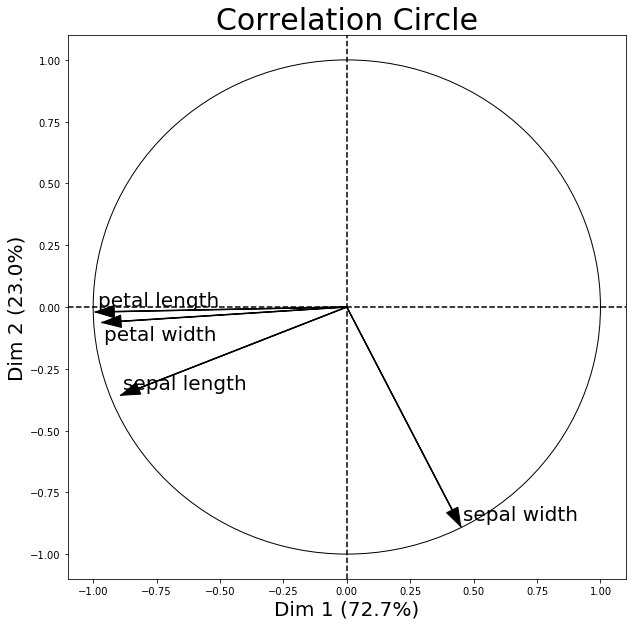# plot_pca_correlation_graph: plot correlations between original features and principal components


A function to provide a correlation circle for PCA.

> from mlxtend.plotting import plot_pca_correlation_graph


In a so called correlation circle, the correlations between the original dataset features and the principal component(s) are shown via coordinates.

## Example

The following correlation circle examples visualizes the correlation between the first two principal components and the 4 original iris dataset features.

• Features with a positive correlation will be grouped together.
• Totally uncorrelated features are orthogonal to each other.
• Features with a negative correlation will be plotted on the opposing quadrants of this plot.
from mlxtend.data import iris_data
from mlxtend.plotting import plot_pca_correlation_graph
import numpy as np

X, y = iris_data()

X_norm = X / X.std(axis=0) # Normalizing the feature columns is recommended

feature_names = [
'sepal length',
'sepal width',
'petal length',
'petal width']

figure, correlation_matrix = plot_pca_correlation_graph(X_norm,
feature_names,
dimensions=(1, 2),
figure_axis_size=10)correlation_matrix

Dim 1 Dim 2
sepal length -0.891224 -0.357352
sepal width 0.449313 -0.888351
petal length -0.991684 -0.020247
petal width -0.964996 -0.062786

Further, note that the percentage values shown on the x and y axis denote how much of the variance in the original dataset is explained by each principal component axis. I.e.., if PC1 lists 72.7% and PC2 lists 23.0% as shown above, then combined, the 2 principal components explain 95.7% of the total variance.

## API

plot_pca_correlation_graph(X, variables_names, dimensions=(1, 2), figure_axis_size=6, X_pca=None, explained_variance=None)

Compute the PCA for X and plots the Correlation graph

Parameters

• X : 2d array like.

The columns represent the different variables and the rows are the samples of thos variables

• variables_names : array like

Name of the columns (the variables) of X

dimensions: tuple with two elements. dimensions to be plotted (x,y)

figure_axis_size : size of the final frame. The figure created is a square with length and width equal to figure_axis_size.

• X_pca : np.ndarray, shape = [n_samples, n_components].

Optional. X_pca is the matrix of the transformed components from X. If not provided, the function computes PCA automatically using mlxtend.feature_extraction.PrincipalComponentAnalysis Expected n_componentes >= max(dimensions)

• explained_variance : 1 dimension np.ndarray, length = n_components

Optional. explained_variance are the eigenvalues from the diagonalized covariance matrix on the PCA transformatiopn. If not provided, the function computes PCA independently Expected n_componentes == X.shape

Returns

matplotlib_figure, correlation_matrix

Examples

For usage examples, please see http://rasbt.github.io/mlxtend/user_guide/plotting/plot_pca_correlation_graph/